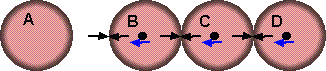# Physics - multiple simultaneous collisions

My first thought is that if three items are moving around then the probability of a single collision in a time interval would be proportional to the the length of the time interval. Therefore as the time interval is made infinitesimally small the probability of a multiple collision would seem to be infinitely small.

If our animation is being broken up into finite time intervals and if a multiple collision is detected in a time interval then, one way to handle this might be to subdivide the time interval until the multiple collision can be resolved into a sequence of two-way collisions.

However multiple collisions do not always happen like this. It may that are a number of stationary, touching objects which are then hit by a moving object. In this case we get a network of impulses similar to a framework of static forces. Like forces, impulses happen between two objects only and Newtons laws have equivalent for impulses.

## Example 1

The following example was given to me from the Open Forum discussion here.Imagine A,B,C and D are pool balls. B,C and D are initially stationary and touching to form a triangle. Ball B is then hit by ball A, assuming that ball A is initially travelling at 1 m/s towards the right va=(1,0,0), all balls weigh 0.1 Kg, friction can be ignored and all collisions are perfectly elastic. What is the final speed of all balls?

When ball A hits ball B then I think that there will be a set of instantaneous impulses setup between pairs of balls shown by pairs of black arrows on the above diagram.

I am assuming that these impulses act normal to the surfaces where the balls are touching, I'm not sure this would be true in practice, perhaps all the impulses should all be horizontal, in which case there is a anticlockwise spin imparted to ball B and a clockwise spin imparted to ball C?

Anyway I will assume the impulses are normal for now.

These impulses have to push against something and this is the inertia due to the mass of the balls, this is shown by the blue arrows.

It seems to me that we should be able to solve this 'network' of impulses in the same way that we could solve a set of forces in a static framework of struts.

I am not sure how to take it from here can anyone help me?

I think we could setup a set of simultaneous equations to solve this, but here are may possible solutions which conserve momentum and energy. For instance B could be stationary after the collision.

## Example 2When we are stuck it sometimes helps to take a simpler example.

In this example after A hits B then A,B and C will remain stationary and D continues on at the same velocity as A was originally travelling.

But is this the only possible outcome that conserves energy and momentum? If not what other criteria are involved to determine the final velocities?

Where I can, I have put links to Amazon for books that are relevant to the subject, click on the appropriate country flag to get more details of the book or to buy it from them.

Commercial Software Shop

Where I can, I have put links to Amazon for commercial software, not directly related to the software project, but related to the subject being discussed, click on the appropriate country flag to get more details of the software or to buy it from them.Dark Basic Professional Edition - It is better to get this professional editionThis is a version of basic designed for building games, for example to rotate a cube you might do the following: make object cube 1,100 for x=1 to 360 rotate object 1,x,x,0 next x

This site may have errors. Don't use for critical systems.